## Direct proof of a theorem about compact funcoids ★★

Author(s): Porton

Conjecture   Letis a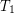-separable (the same as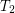for symmetric transitive) compact funcoid and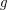is a uniform space (reflexive, symmetric, and transitive endoreloid) such that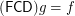. Then.

The main purpose here is to find a direct proof of this conjecture. It seems that this conjecture can be derived from the well known theorem about existence of exactly one uniformity on a compact set. But that would be what I call an indirect proof, we need a direct proof instead.

The direct proof may be constructed by correcting all errors an omissions in this draft article.

Direct proof could be better because with it we would get a little more general statement like this:

Conjecture   Letbe a-separable compact reflexive symmetric funcoid andbe a reloid such that
\item; \item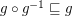.

Then.

## Generalized path-connectedness in proximity spaces ★★

Author(s): Porton

Letbe a proximity.

A setis connected regardingiff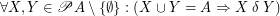.

Conjecture   The following statements are equivalent for every endofuncoidand a set:
\itemis connected regarding. \item For every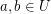there exists a totally ordered set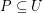such that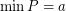,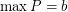, and for every partion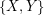of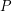into two sets,such that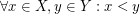, we have.

Keywords: connected; connectedness; proximity space

## Dirac's Conjecture ★★

Author(s): Dirac

Conjecture   For every setofpoints in the plane, not all collinear, there is a point incontained in at leastlines determined by, for some constant.

Keywords: point set